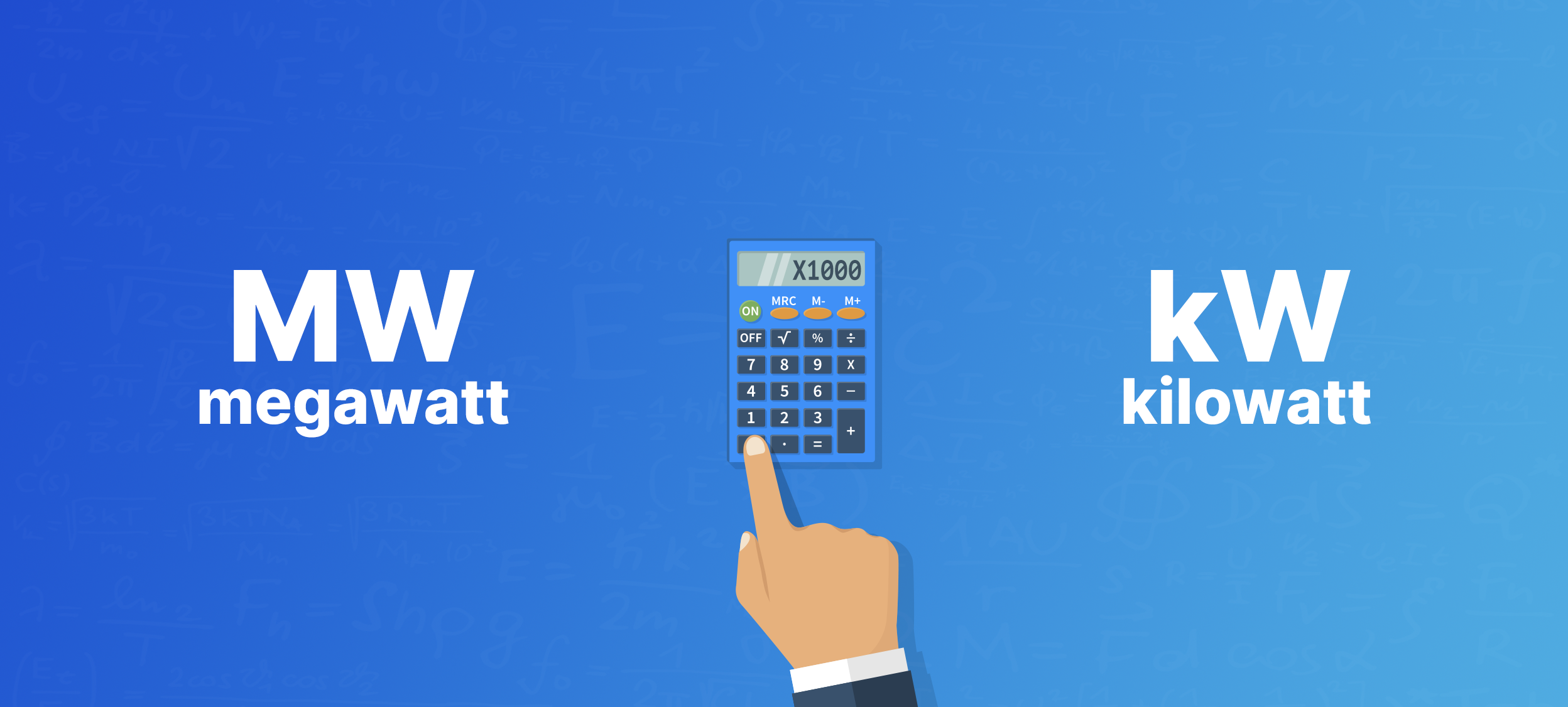# What are megawatts & kilowatts, how do you convert them?

UpdatedYour go-to guide for converting kilowatts and megawatts.

If you just started researching solar panels, you might be overwhelmed by all of the solar jargon you’ve come across. What even is a kilowatt, and why are solar system sizes always described in them? What about megawatts?

Don’t worry - we’ve got you covered. We’ve broken down what you need to know about converting megawatts to kilowatts, and how these units relate to you and your solar system.

### Megawatts to kilowatts at a glance:

• One kilowatt (kW) is equal to 1,000 watts
• One megawatt (MW) is equal to 1,000 kilowatts (or in other words, 1,000,000 watts)
• Convert kilowatts to megawatts by dividing the number of kilowatts by 1,000
• Convert megawatts to kilowatts by multiplying the number of megawatts by 1,000
• Residential solar panel systems are typically measured in kilowatts

## Megawatt to kilowatt conversion table

Remember: one megawatt is equal to 1,000 kilowatts.

Here’s a quick conversion chart to help you convert megawatts to kilowatts:

Table 1. MW to kW conversion table
Megawatt (MW) Kilowatt (kW)
0.01 MW 10 kW
0.1 MW 100 kW
1 MW 1,000 kW
5 MW 5,000 kW
10 MW 10,000 kW
15 MW 15,000 kW
20 MW 20,000 kW
100 MW 100,000 kW
1,000 MW 1,000,000 kW

### How to convert megawatts to kilowatts

Converting megawatts to kilowatts is easy! All you have to do is multiply the number of megawatts by 1,000:

Number of megawatts x 1,000 = Number of kilowatts

Here’s an example of how to find how many kilowatts are in 30 megawatts:

30 MW x 1,000 = 30,000 kW

If you want to go from kilowatts to megawatts, do the opposite! Simply divide the number of kilowatts by 1,000 to find how many megawatts you have:

Number of kilowatts ÷ 1,000 = Number of megawatts

## What even are megawatts and kilowatts?

A watt is the basic unit of power, and represents the rate at which energy flows through an electrical system.

Watts are a pretty small unit of measure, and most households use thousands of watts - so instead of listing out all those zeros, you convert to kilowatts or megawatts.

A kilowatt is equal to 1,000 watts. So, if your home required 7,000 watts of power, you could also write it as 7 kW.

One megawatt is equal to one million watts. Megawatts are much larger than kilowatts, and typically describe power at a large scale, like how much power an entire utility company is delivering.

After megawatts, you get to gigawatts, which you would use to describe the amount of power generated on a country-wide scale. Gigawatts are equal to one billion watts!

### Are kilowatts and kilowatt-hours different?

Yes, kilowatts and kilowatt-hours are two different things

Kilowatts represent power. A kilowatt-hour (kWh) measures energy, or the amount of power over time. You’re probably familiar with kilowatt-hours - it’s the unit used to measure your energy usage on your electricity bill.

#### How to figure out how many kWh an appliance uses

To find out how many kilowatt-hours an appliance is using, you just need to multiply the wattage of the appliance (which tells you how much power it needs to run) by the number of hours it’s running.

Let’s use a 3.5 kW air conditioner that runs for 5 hours as an example:

3.5 kW x 5 hours = 17.5 kWh

Megawatt-hours work the same way. Take the number of megawatts and multiply it by the hours the system is running. Again, megawatts represent a very large amount of power, so you probably won’t have to deal with them too often.

## Why are solar panel systems sold in kilowatts?

All solar panels come with a power output wattage that represents how much power the panel can provide under ideal conditions. Most panels today have a power output rating between 350 watts and 400 watts.

You need more than one solar panel to power your home, which means you’re going to end up having thousands of watts’ worth of solar on your roof. So, instead of saying your system is 7,500 watts in size, we convert it to kilowatts - 7.5 kW.

Because all panels have a rated wattage, the pricing for solar panels is usually listed as the “cost per watt”. This allows for uniform pricing that can easily be compared from system to system.

## How many kilowatts of solar should you install on your roof?

Most home solar systems today are between 6 kW and 7 kW in size. But the exact number you’ll need depends on factors like your energy usage, where you live, the size of your roof, even the direction your house faces!

The easiest way to figure out how many kilowatts of solar you need is by using our state-of-the-art solar panel calculator. Our calculator not only tells you what system size you need, but also what kind of solar savings you can expect!#### Andrew Sendy

##### Home Solar Journalist

Andy is deeply concerned about climate change but is also concerned about cost of living pressures on American families. He advocates for solar energy and solar battery storage only to the extent that they make financial sense for homeowners. He is not affiliated with any particular solar company in the United States.